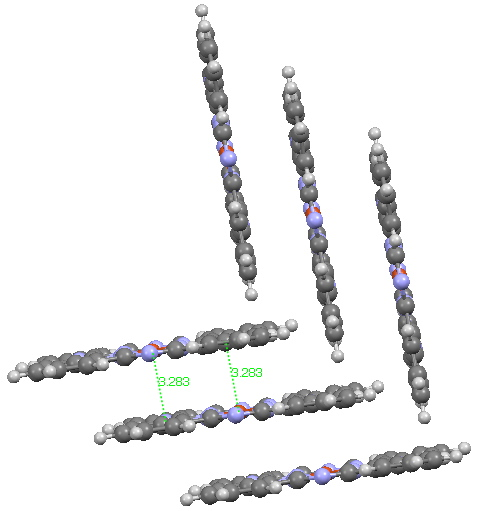## The colour of Monastral blue (part 2).

Andy Mclean posted a comment to my story of copper phthalocyanine (Monastral blue). The issue was its colour, and more specifically why this pigment has two peaks λmax 610 and 710nm making it blue. The first was accurately reproduced by calculation on the monomer, but the second was absent with such a model. Andy suggested this latter was due to stacking. Here, the calculated spectrum of a stacked dimer is explored.Copper phthalocyanine, showing herring-bone stacking. Click for 3D.

The X-ray structure (above) shows layers of the phthalocyanine, dislocated so that the Cu of one unit aligns perfectly with a N of the units above and below the first one (Cu-N 3.28Å). This corresponds to the di-axially solvated system I explored in a comment appended to the original post. The TD-DFT calculated (since each unit is a doublet radical, the dimer was treated as a triplet state, this being much lower in energy than a singlet closed shell state)  electronic spectrum for two units, stacked above each other as shown above reveals two transitions at ~ 600 and  620 nm. This is still some way away from reproducing the measured  (solid state or solution spectra).The hypothesis must now be that the effect of such π-π stacking on the electronic spectrum converges only slowly with the degree of stacking (if indeed it is that that is the root cause of the 710nm transition). A calculation on a triple-layered model is currently under way (this being the absolute limit of what can be done without a periodic boundary model). The spectrum will be appended to this post in a week or so (see below). There is little sign of the spectrum evolving a quite separate band at 710nm. The model is still incomplete!